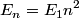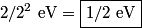## Solution to 1986 Problem 90

 The allowed energies in an infinite square well are\begin{align*}E_n = E_1 n^2\end{align*}The wavefunction shown corresponds to n = 2 because it has two local extrema. Therefore, the wavefunction of the ground state is$2/2^2 \mbox{ eV} = \boxed{1/2 \mbox{ eV}}$. Therefore, (C) is the correct answer.